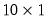Coef
Coefficient vector. Coefficients are used to represent the parameters of equations and systems.
Coef Declaration
coef declare coefficient vector.
There are two ways to create a coef. First, enter the coef keyword, followed by a name to be given to the coefficient vector. The dimension of the coef may be provided in parentheses after the keyword:
coef alpha
coef(10) beta
If no dimension is provided, the resulting coef will contain a single element.
You may also combine a declaration with an assignment statement. If you do not provide an explicit assignment statement, a new coef vector will be initialized to zero.
See also param for information on initializing coefficients, and the entries for each of the estimation objects ( Equation, Logl, Pool, Sspace, System, and Var) for additional methods of accessing coefficients.
Coef Views
display display table, graph, or spool in object window.
label label view.
sheet spreadsheet view of the coefficient.
stats descriptive statistics.
Coef Procs
clearcollabels clear the column label in a coef object.
clearhist clear the contents of the history attribute.
clearremarks clear the contents of the remarks attribute.
clearrowlabels clear the row labels in a coef object.
copy creates a copy of the coef.
displayname set display name.
export save as Excel 2007 XLSX, CSV, tab-delimited ASCII text, RTF, HTML, Enhanced Metafile, PDF, TEX, or MD file on disk.
fill fill the elements of the coefficient vector.
import imports data from a foreign file into the object.
read import data into coefficient vector.
resize resize the coef object.
setattr set the value of an object attribute.
setcollabels set the column label in a coef object.
setformat set the display format for the coefficient vector spreadsheet.
setindent set the indentation for the coefficient spreadsheet.
setjust set the horizontal justification for all cells in the spreadsheet view of the coef object.
setrowlabels set the row labels in a coef object.
setwidth set the column width for the coefficient spreadsheet.
showlabels displays the custom row and column labels of a coef spreadsheet.
write export data from coefficient vector.
Coef Graph Views
Graph creation views are discussed in detail in “Graph Creation Command Summary”.
area area graph.
bar bar graph.
boxplot boxplot graph.
distplot distribution graph.
dot dot plot graph.
line line graph.
qqplot quantile-quantile graph.
seasplot seasonal line graph.
spike spike graph.
Coef Data Members
@attr("arg") string containing the value of the arg attribute, where the argument is specified as a quoted string.
@collabels string containing the column label of the coef.
@description string containing the Coef object’s description (if available).
@detailedtype string describing the object type: “COEF”.
@displayname string containing the Coef object’s display name. If the Coef has no display name set, the name is returned.
@droprow(arg) Returns the Coef with the rows defined by arg removed. arg may be an integer, vector of integers, string, or svector of strings. Integer values correspond to rows and string values correspond to previously defined row labels.
@name string containing the Coef object’s name.
@remarks string containing the Coef object’s remarks (if available).
@row(arg) Returns the rows defined by arg. arg may be an integer, vector of integers, string, or svector of strings. Integer values correspond to rows and string values correspond to previously defined row labels.
@rowlabels string containing the row labels of the coef.
@rows number of rows in the coef.
@type string describing the object type: “COEF”.
@updatetime string representation of the time and date at which the Coef was last updated.
(i) i-th element of the coefficient vector. Simply append “(i)” to the coef name (without a “.”).
Coef Examples
The coefficient vector declaration:
coef(10) coef1=3
creates a 10 element coefficient vector COEF1, and initializes all values to 3.
Suppose MAT1 is amatrix, and VEC1 is a 20 element vector. Then:
coef mycoef1=coef1
coef mycoef2=mat1
coef mycoef3=vec1
create, size, and initialize the coefficient vectors MYCOEF1, MYCOEF2 and MYCOEF3.
Coefficient elements may be referred to by an explicit index. For example:
vector(10) mm=beta(10)
scalar shape=beta(7)
fills the vector MM with the value of the tenth element of BETA, and assigns the seventh value of BETA to the scalar SHAPE.